UPSC  >  NCERT Summary: Summary of Physics- 2

# NCERT Summary: Summary of Physics- 2 | Science & Technology for UPSC CSE

 Table of contentsLightReflection, Refraction & Dispersion of Light1. Reflection of Light2. Refraction of Light3. Dispersion of LightMagnetism, Electricity & Electromagnetism1. Magnetism2. Electricity3. Electromagnetism1 Crore+ students have signed up on EduRev. Have you?

Light

Light or visible light is electromagnetic radiation within the portion of the electromagnetic spectrum that can be perceived by the human eye.
• To understand light you have to know that what we call light is what is visible to us. Visible light is the light that humans can see. Other animals can see different types of light. Dogs can see only shades of gray and some insects can see light from the ultraviolet part of the spectrum.
• As far as we know, all types of light move at one speed when in a vacuum. The speed of light in a vacuum is 299,792,458 meters per second.

Medium of Propagation of Light

• Any medium through which light can travel is an optical medium.
• If this medium is such that light travels with equal speed in all directions, then the medium is called a homogeneous medium.
• The homogeneous media through which light can pass easily, are called transparent media.
• The media through which light cannot pass, are called opaque media. Again the media through which light can pass partly, are called translucent media.

Reflection, Refraction & Dispersion of Light

1. Reflection of Light

When a ray of light approaches a smooth polished surface and the light ray bounces back, it is called the reflection of light.
• Light travels along a Straight Line: Light is reflected from all surfaces. Regular reflection takes place when light is incident on smooth, polished, and regular surfaces.
• Incident Ray: After striking the surface, the ray of light is reflected in another direction. The light ray, which strikes any surface, is called the incident ray. The ray that comes back from the surface after reflection is known as the reflected ray.
• The angle of incidence: The angle between the normal and incident ray is called the angle of incidence.
• The angle of reflection: The angle between the normal and the reflected ray is known as the angle of reflection.

Laws of Reflection of Light

• Two laws of reflection are:
(i) The angle of incidence is equal to the angle of reflection.
(ii) Incident ray, reflected ray, and the normal drawn at the point of incidence to the reflecting surface, lie in the same plane.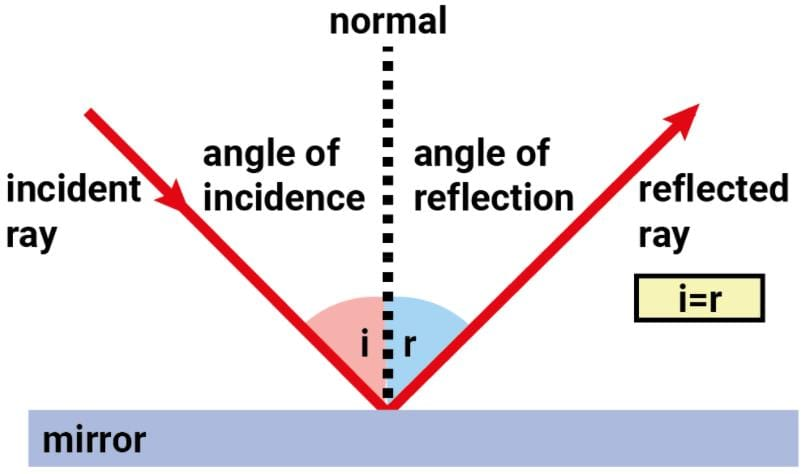• Irregular reflection: When all the parallel rays reflected from a plane surface are not parallel, the reflection is known as diffused or irregular reflection.
• Regular reflection: On the other hand reflection from a smooth surface like that of a mirror is called regular reflection.
Types of Images after Reflection
• When rays of light coming from a point of source, after reflection or refraction, actually meet at another point or appear to diverge from another point, the second point is called the image of the first point.
Images may be of two types:
(i) Real
(ii) Virtual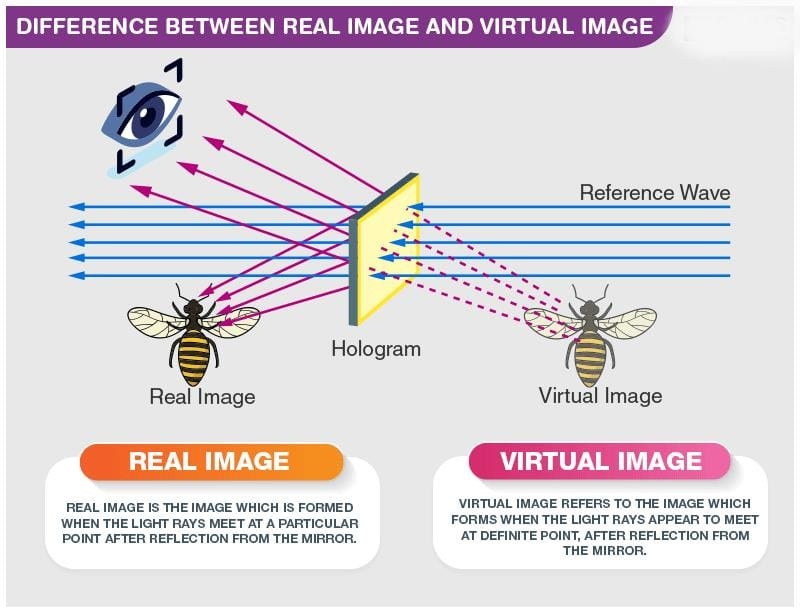• Real Image: An image that can be obtained on a screen is called a real image.
• Virtual Image: An image that cannot be obtained on a screen is called a virtual image.
• The image formed by a plane mirror is erect. It is virtual and is of the same size as the object. The image is at the same distance behind the mirror as the object is in front of it.
Types of Mirrors
• The reflecting surface of a spherical mirror may be curved inwards or outwards.

Concave Mirror:

A spherical mirror, whose reflecting surface is curved inwards, that is, faces towards the center of the sphere, is called a concave mirror.

• Concave mirrors are commonly used in torches, search-lights, and vehicle headlights to get powerful parallel beams of light.
• They are often used as shaving mirrors to see a larger image of the face. The dentists use concave mirrors to see large images of the teeth of patients.
• Large concave mirrors are used to concentrate sunlight to produce heat in solar furnaces.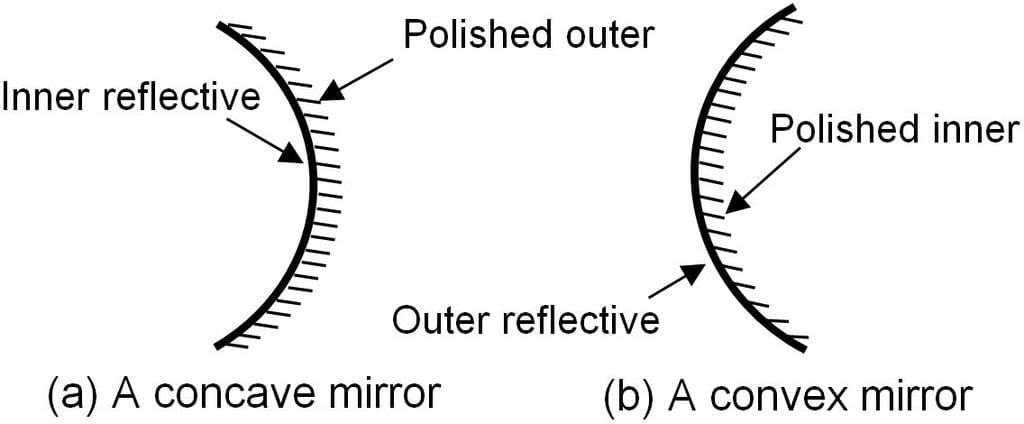Convex Mirror

A spherical mirror whose reflecting surface is curved outwards is called a convex mirror.

• Convex mirrors are commonly used as rear-view (wing) mirrors in vehicles.
• These mirrors are fitted on the sides of the vehicle, enabling the driver to see traffic behind him/her to facilitate safe driving.
• Convex mirrors are preferred because they always give an erect, though diminished, image.
• Also, they have a wider field of view as they are curved outwards. Thus, convex mirrors enable the driver to view much larger area than would be possible with a plane mirror.

Other Terms Involved

Pole: The center of the reflecting surface of a spherical mirror is a point called the pole. It lies on the surface of the mirror. The pole is usually represented by the letter P.

Center of Curvature: The reflecting surface of a spherical mirror forms a part of a sphere. This sphere has a center. This point is called the center of curvature of the spherical mirror. It is represented by the letter C.

Note: The center of curvature is not a part of the mirror. It lies outside its reflecting surface. The center of curvature of a concave mirror lies in front of it. However, it lies behind the mirror in the case of a convex mirror.

Radius of Curvature (R): The radius of the sphere of which the reflecting surface of a spherical mirror forms a part is called the radius of curvature of the mirror. It is represented by the letter R. You may note that the distance PC is equal to the radius of curvature.

Principal Axis: Imagine a straight line passing through the pole and the center of curvature of a spherical mirror. This line is called the principal axis.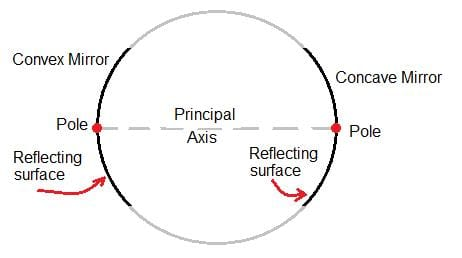Spherical Mirror

Types of Lenses

• Concave Lens: Those lenses which feel thicker in the middle than at the edges are convex lenses. Those which feel thinner in the middle than at the edges are concave lenses. Notice that the lenses are transparent and light can pass through them.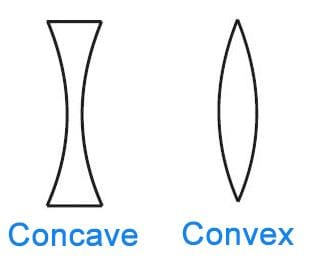Concave and Convex Lens
• Convex Lens: A convex lens converges (bends inward) the light generally falling on it. Therefore, it is called a converging lens. On the other hand, a concave lens divergess (bends outward) the light and is called a diverging lens.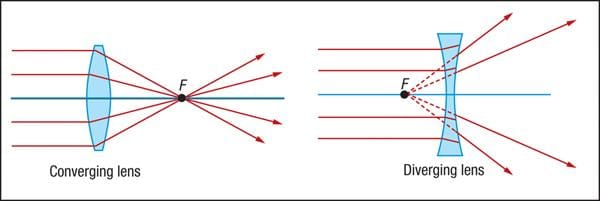Types of Images formed by Lenses
• Image formed by Concave Lens: A concave lens always forms an erect, virtual, and smaller image than the object.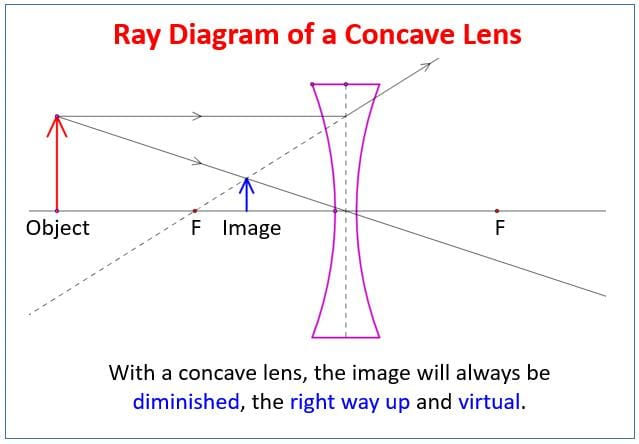• Image formed by Convex Lens: A convex lens can form a real and inverted image. When the object is placed very close to the lens, the image formed is virtual, erect, and magnified. When used to see objects magnified, the convex lens is called a magnifying glass.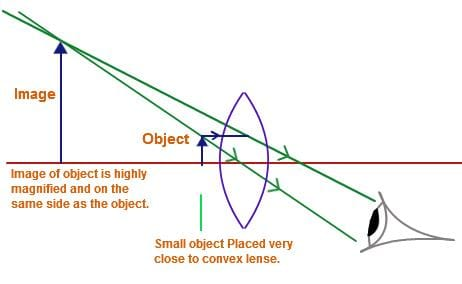The convex lens as a magnifying glass

Other Terms Involved

• Principal Axis: The two surfaces of the lens are parts of two spheres. The straight line joining obtained by joining two centers of the spheres is called the Principal axis. Generally, we use lenses whose surfaces have equal curvature. In such lenses, if we take a point on the principal axis inside the lens equidistant from the two surfaces, the point is called the optical center of the lens.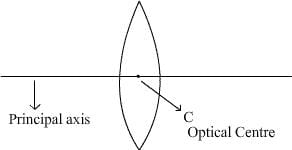Optical center and principal axis of a convex lens
• Focus of Convex Lens: If a beam of parallel rays, traveling parallel to the principal axis of a convex lens, is refracted by the lens, the rays become converging and intersect each other at a particular point of the axis. The point is called the focus of the convex lens.
• Focal Length of Lens: The focal length of a lens is the distance between the optical center and the focus of the lens.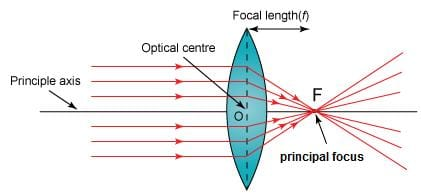Classification of solids on the basis of electricity conduction
• Power of Lens: The power of a lens is a measure of the degree of convergence (in the case of a convex lens) or divergence (in the case of a concave lens). It is defined as the reciprocal of its focal length expressed in meters.
• S.I. Unit of power of a lens: Dioptre, the symbol being D. Thus, 1 dioptre is the power of a lens whose focal length is 1 meter. 1D = 1m–1
• You may note that the power of a convex lens is positive and that of a concave lens is negative.

2. Refraction of Light

The phenomenon due to which a ray of light deviates from its path, at the surface of separation of two media, when the ray of light is traveling from one optical medium to another optical medium is called refraction of light.

When a ray of light travels from an optically rare medium to an optically denser medium.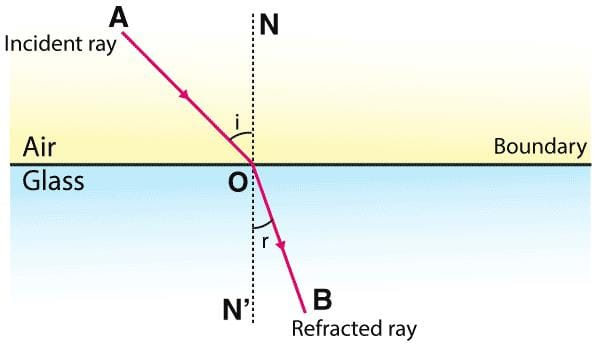Refraction of Light

Phenomenon of Refraction

• When a ray of light travels from an optically denser medium to an optically rare medium, it bends away from the normal at the surface of the separation of two media.
• When a ray of light strikes the surface of separation of two media normally, it does not deviate from its original path. Some indexes of refraction are diamond (2.419), glass (1.523), and water (1.33).
• Total internal reflection: It is the phenomenon that involves the reflection of all the incident light off the boundary.
Total internal reflection only takes place when both of the following two conditions are met:
(i) The light is in the more dense medium and approaching the less dense medium.
(ii) The angle of incidence is greater than the so-called critical angle.
• Total internal reflection will not take place unless the incident light is traveling within the more optically dense medium towards the less optically dense medium.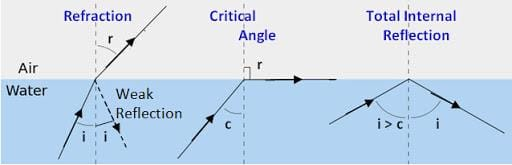The Phenomenon of Total Internal Reflection

3. Dispersion of Light

It is the phenomenon splitting of a beam of white light into its constituent colors on passing through a prism.

• The order of colors from the lower end are violet, indigo, blue, green, yellow, orange, and red
• At one end of the band, there is red and at the other violet.
• The sequence of colors can be best remembered by the word VIBGYOR’ which is formed by taking the initial letter of each color.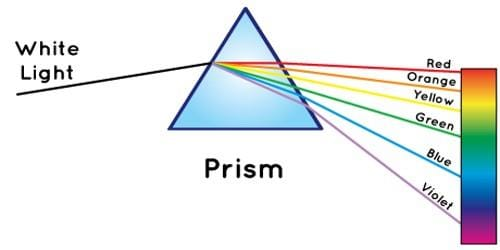Dispersion of Light
• A laser is just a really powerful beam of light. The laser isn’t a word but an acronym. It stands for Light Amplification By Stimulated Emission Of Radiation.
Magnetism, Electricity & Electromagnetism

1. Magnetism

Magnetism is a  physical phenomenon produced by the motion of electric charge, which results in attractive and repulsive forces between objects.

• The word magnet is derived from the name of an island in Greece called Magnesia where magnetic ore deposits were found, as early as 600 BC. Magnetite, an iron ore, is a natural magnet. It is called a lodestone.
• When a bar magnet is freely suspended, it points in the north-south direction. The tip which points to the geographic north is called the north pole and the tip which points to the geographic south is called the south pole of the magnet. There is a repulsive force when the north poles (or south poles) of two magnets are brought close together. Conversely, there is an attractive force between the north pole of one magnet and the south pole of the other.
• The properties of a magnet are:
(i) It attracts a small piece of iron towards it.
(ii) It always comes to rest in a north-south direction when suspended freely.
(iii) As poles repel, unlike poles attracts each other
(iv) Magnetic poles always exist in pairs.
(v) The strength of a magnet is maximum at poles located near the poles.
• The phenomenon due to which an unmagnetized magnetic substance behaves like a magnet, due to the presence of some other magnet, is called magnetic induction. Magnetic induction takes place first then magnetic attraction.

Magnetic Induction

• Magnetic induction depends upon the nature of the magnetic substance. Magnetic induction is inversely proportional to the distance between the inducing magnet and the magnetic substance. The more powerful the inducing magnet, the more strong will be the magnetism in magnetic substance.

Magnetic Field

• The space around the magnet where its influence can be detected is called the magnetic field.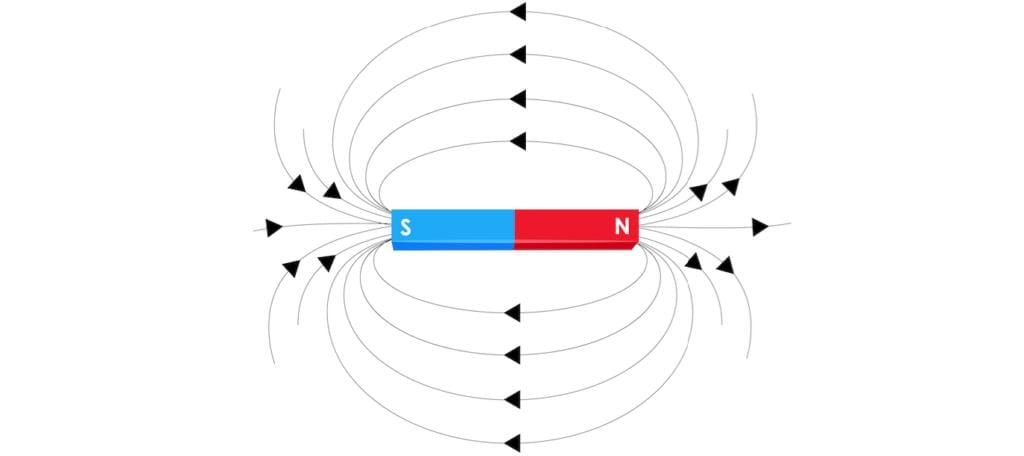Magnetic Field Around the Bar Magnet
• A curve in a magnetic field, along with a free north magnetic pole will move, is called the magnetic line of force. The direction of magnetic lines of force is the direction in which the free north pole will move in a magnetic field.
(i) They travel from north to south pole outside the magnet and from south to north pole inside the magnet.
(ii) They mutually repel each other.
(iii) They never intersect with each other.
• The earth behaves as a magnet with the magnetic field pointing approximately from the geographic south to the north. At a particular place on earth, the magnetic north is not usually in the direction of the geographic north. The angle between the two directions called declination.

2. Electricity

The phenomenon due to which a suitable combination of bodies on rubbing, get electrified is called electricity. If a charge on a body is not allowed to flow, it is called static electricity.

• Matters are made of atoms. An atom is basically composed of three different components — Electrons, Protons, and Neutrons. An electron can be removed easily from an atom. When two objects are rubbed together, some electrons from one object move to another object.
Example: When a plastic bar is rubbed with fur, electrons will move from the fur to the plastic stick. Therefore, plastic bar will be negatively charged and the fur will be positively charged.
• When two objects are rubbed together, some electrons from one object move to another object.
Example: When a plastic bar is rubbed with fur, electrons will move from the fur to the plastic stick. Therefore, plastic bar will be negatively charged and the fur will be positively charged.
• When you bring a negatively charged object close to another object, electrons in the second object will be repelled from the first object. Therefore, that end will have a negative charge. This process is called charging by induction.
• When a negatively charged object touches a neutral body, electrons will spread on both objects and make both objects negatively charged. This process is called charging by conduction. The other case, positively charged object touching the neutral body, is just the same in principle.

Types of Substance

• Substances can be classified into three typesInsulators, Conductors, and Semiconductors.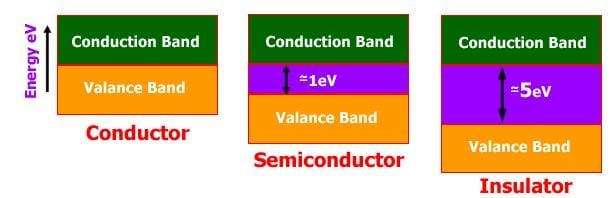Classification of Solids on the basis of Electricity Conduction
• Conductors are materials in which electrical charges and heat energy can be transmitted very easily. Almost all metals such as gold, silver, copper, iron, and lead are good conductors.
(i) Insulators are materials that allow very little electrical charges and heat energy to flow. Plastics, glass, dry air, and wood are examples of insulators.
(ii) Semiconductors are materials that allow the electrical charges to flow better than insulators, but less than conductors.
Example: Silicon and Germanium.

Types of Electric Charges

• There are two different types of electric charges namely the positive and negative charges. Like charges repel and unlike charges attract each other.
• Electric current always flows from the point of high potential. The potential difference between two conductors is equal to the work done in conducting a unit positive charges from one conductor to the other conductor through a metallic wire.
• The flow of charge is called the current and it is the rate at which electric charges pass through a conductor. The charged particle can be either positive or negative. In order for a charge to flow, it needs a push (a force) and it is supplied by voltage or potential difference. The charge flows from high potential energy to low potential energy.
• A closed-loop of current is called an electric circuit. The current [I] measures the amount of charge that passes a given point every second. The unit for current is Ampere [A]. 1 A means that 1 C of charge passes every second.
• When current flows through a conductor it offers some obstruction to the flow of current The obstruction offered to flow of current by the conducting wire is called its resistance in the passage of electricity.
• The unit of resistance is Ohm. The resistance varies in different materials.
For example gold, silver, and copper have low resistance, which means that current can flow easily through these materials. Glass, plastics, and wood have very high resistance, which means that current can not pass through these materials easily.

3. Electromagnetism

The branch of physics which deals with the relationship between electricity and magnetism is called electromagnetism.

• Whenever current is passed through a straight conductor it behaves like a magnet. The magnitude of magnetic effect increases with the increase in the strength of current.
• Faraday’s law of induction is one of the important concepts of electricity. It looks at the way changing magnetic fields can cause current to flow in wires. Basically, it is a formula/concept that describes how potential difference (voltage difference) is created and how much is created. It’s a huge concept to understand that the changing of a magnetic field can create voltage.
• He discovered that the changes in the magnetic field and the size of the field were related to the amount of current created. Scientists also use the term magnetic flux. Magnetic flux is a value that is the strength of the magnetic field multiplied by the surface area of the device.

Coulomb’s Law

The law states that the magnitude of the electrostatic force of attraction or repulsion between two point charges is directly proportional to the product of the magnitudes of charges and inversely proportional to the square of the distance between them
• Coulomb’s Law is one of the basic ideas of electricity in physics. The law looks at the forces created between two charged objects.
• As distance increases, the forces and electric fields decrease. This simple idea was converted into a relatively simple formula. The force between the objects can be positive or negative depending on whether the objects are attracted to each other or repelled.
• When you have two charged particles, an electric force is created. If you have larger charges, the forces will be larger. If you use those two ideas, and add the fact that charges can attract and repel each other you will understand Coulomb’s Law.
• It’s a formula that measures the electrical forces between two objects.

F = kq1q/ r2

» Where ”F” is the resulting force between the two charges.
» The distance between the two charges is ”r”. The “r” actually stands for “radius of separation” but you just need to know it is a distance.
» The ”q2" and ”q2" values for the amount of charge in each of the particles. Scientists use Coulombs as units to measure charge.
» The constant of the equation is “k”

Types of Current- AC & DC

• There are two main types of current in our world. One is direct current (DC) which is a constant stream of charges in one direction. The other is alternating current (AC) that is a stream of charges that reverses direction.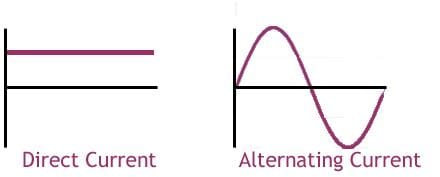• The current in DC circuits is moving in a constant direction. The amount of current can change, but it will always flow from one point to another. In alternating current, the charges move in one direction for a very short time, and then they reverse direction. This happens over and over again.

MECHANICS

• Motion: In physics, motion is change of location or position of an object with respect to time. Mechanical motion is of two types, transitional (linear) and rotational (spin).
• SPEED: The speed of a moving body is the rate at which it covers distance i.e. the distance it covers per unit of time.

Speed: (distance travelled/ time required.) The S.I. Unit of speed is ms.

VELOCITY: The distance covered by an object in a specified direction in unit time interval is called velocity. The S.I. Unit of velocity is m/s.

• Average velocity can be calculated by dividing displacement over time.
• The instantaneous velocity shows the velocity of an object at one point.
• The difference between speed and velocity is: Speed is the distance travelled by an object in a particular time. Velocity is the speed in a particular direction.
• ACCELERATION: When an object’s velocity changes, it accelerates. Acceleration shows the change in velocity in a unit time. Velocity is measured in meters per second, m/s, so acceleration is measured in (m/s)/ s, or m/s2, which can be both positive and negative. The symbol for acceleration is a (boldface).
• When the velocity decreases the body is said to undergo retardation or deceleration.
• Acceleration Due to Gravity: Galileo was the first to find out that all objects falling to Earth have a constant acceleration of 9.80 m/s2 regardless of their mass. Acceleration due to gravity is given a symbol g, which equals to 9.80 m/s2.
• FORCE: Force can be defined as a push or a pull. (Technically, force is something that can accelerate objects.) Force is measured by N (Newton). A force that causes an object with a mass of 1 kg to accelerate at 1 m/s is equivalent to 1 Newton.
• Newton’s law of universal gravitation states that every massive particle in the universe attracts every other massive particle with a force which is directly proportional to the product of their masses and inversely proportional to the square of the distance between them.
• In equation form, the gravitational force F = G(m1 m2)/ r2 where r is the distance between two bodies of masses mand m2 and G the universal gravitational constant.
• Centripetal Force: For a body to move in a circle there must be a force on it directed towards the centre. This is called the centripetal force and is necessary to produce continuous change of direction in a circular motion.
• The magnitude of the centripetal force on an object of mass m moving at a speed v along a path with radius of curvature r is given by the relation F =mv2/r The direction of the force is toward the center of the circle in which the object is moving. Centrifugal force is equal and opposite to centripetal force, i.e it acts outwards.
• WEIGHT: the weight of a body is the force with which the earth attracts the body towards its centre. The weight of a body should not be confused with its mass, which is a measure of the quantity of matter contained in it. Mass shows the quantity, and weight shows the size of gravity. The weight of a body is maximum at the poles and minimum at equator.
• If you know your mass, you can easily find your weight because W = mg where:

W is weight in Newton (N),
m is mass in kg, and
g is the acceleration of gravity in m/s2.

• Weight is measured by Newton (N).
• It is now obvious that the value of g is maximum at poles and minimum at equator. At the centre of earth, g would be zero.
• It should be noted here that on the surface of the moon the value of the acceleration due to gravity is neraly one-sixth of that on earth, and therefore, an object on the moon would weigh only one-sixth its weight on earth.
• Newton’s Laws of Motion:

1. Newtons First Law of Motion:

• Newton’s first law of motion states that “An object at rest tends to stay at rest and an object in motion tends to stay in motion with the same speed and in the same direction unless acted upon by an unbalanced force.” . Every object in a state of uniform motion tends to remain in that state of motion unless an external force is applied to it.
• In fact, it is the natural tendency of objects to resist changes in their state of motion. This tendency to resist changes in their state of motion is described as inertia.
• Inertia: Inertia is the tendency of an object to resist changes in its state of motion. But what is meant by the phrase state of motion? The state of motion of an object is defined by its velocity - the speed with a direction. Thus, inertia could be redefined as follows: Inertia: tendency of an object to resist changes in its velocity.
• There are many more applications of Newton’s first law of motion.
• Blood rushes from your head to your feet while quickly stopping when riding on a descending elevator.
• The head of a hammer can be tightened onto the wooden handle by banging the bottom of the handle against a hard surface.
• While riding a skateboard (or wagon or bicycle), you fly forward off the board when hitting a curb or rock or other object which abruptly halts the motion of the skateboard.

2. Newton’s Second Law of Motion:

• The acceleration of an object as produced by a net force is directly proportional to the magnitude of the net force, in the same direction as the net force, and inversely proportional to the mass of the object.
• The relationship between an object’s mass m, its acceleration a, and the applied force F is F = ma. Acceleration and force are vectors (as indicated by their symbols being displayed in slant bold font); in this law the direction of the force vector is the same as the direction of the acceleration vector.

3. Newton’s Third Law of Motion:

• For every action, there is an equal and opposite reaction.
• The statement means that in every interaction, there is a pair of forces acting on the two interacting objects. The size of the forces on the first object equals the size of the force on the second object. The direction of the force on the first object is opposite to the direction of the force on the second object. Forces always come in pairs - equal and opposite action-reaction force pairs.
• The rocket’s action is to push down on the ground with the force of its powerful engines, and the reaction is that the ground pushes the rocket upwards with an equal force.
• There’s also the example of shooting a cannonball. When the cannonball is fired through the air (by the explosion), the cannon is pushed backward. The force pushing the ball out was equal to the force pushing the cannon back, but the effect on the cannon is less noticeable because it has a much larger mass. That example is similar to the kick when a gun fires a bullet forward.
• Friction: Friction is a force that resists the movement oof one surface over another. The force acts in the opposite direction to the way an object wants to slide. If a car needs to stop at a stop sign, it slows because of the friction between the brakes and the wheels.
• Measures of friction are based on the type of materials that are in contact. Concrete on concrete has a very high coefficient of friction. That coefficient is a measure of how easily one object moves in relationship to another. When you have a high coefficient of friction, you have a lot of friction between the materials.
PROPERTIES OF MATTERS
• Properties of matters: A matter can neither be created nor it can be destroyed but it can be transformed from one state to another. Matter is made of basic building blocks commonly called elements which are 112 in number. The matter is made of only one kind of element then the smallest unit of that element is called an atom. If the matter is made of two or more different elements then the smallest unit of matter is called a molecule.
• Molecule is defined as the smallest unit of matter which has independent existence and can retain complete physical and chemical properties of matters.
• According to kinetic theory of matter:
(i) molecules are in the state of continuous motion in all possible directions and hence they posses  kinetic energy which increases with the gain of heat energy or rise in temperature,
(ii). the molecules always attract each other,
(iii). the force of attraction between the molecules decreases with the increase in intermolecular spaces
• The molecules always attract each other. The force of attraction between the similar kind of molecules is called force of cohesion whereas the force of attraction between different kinds of molecules is called force of adhesion.
• In case of solids, the intermolecular space being very small, so intermolecular forces are very large and hence solids have definite size and shape.
• In case of liquids, the intermolecular space being large, so intermolecular forces are small and hence liquids have definite volume but no definite shape.
• In case of gases, the intermolecular space being very large, so intermolecular forces are extremely small and hence gases have neither a definite volume and nor definite shape.
• A solid has definite shape and size. In order to change (or deform) the shape or size of a body, a force is required. If you stretch a helical spring by gently pulling its ends, the length of the spring increases slightly. When you leave the ends of the spring, it regains its original size and shape. The property of a body, by virtue of which it tends to regain its original size and shape when the applied force is removed, is known as elasticity and the deformation caused is known as elastic deformation.
• However, if you apply force to a lump of putty or mud, they have no gross tendency to regain their previous shape, and they get permanently deformed. Such substances are called plastic and this property is called plasticity. Putty and mud are close to ideal plastics.
• When a force is applied on body, it is deformed to a small or large extent depending upon the nature of the material of the body and the magnitude of the deforming force. The deformation may not be noticeable visually in many materials but it is there. When a body is subjected to a deforming force, a restoring force is developed in the body. This restoring force is equal in magnitude but opposite in direction to the applied force. The restoring force per unit area is known as stress. If F is the force applied and A is the area of cross section of the body, Magnitude of the stress = F/A. The SI unit of stress is N m–2 or pascal (Pa). Stress is the restoring force per unit area and strain is the fractional change in dimension.
• HOOKE’S LAW: Robert Hooke, an English physicist (1635 - 1703 A.D) performed experiments on springs and found that the elongation (change in the length) produced in a body is proportional to the applied force or load. In 1676, he presented his law of elasticity, now called Hooke’s law. For small deformations the stress and strain are proportional to each other. This is known as Hooke’s law. Thus, stress ”” strain or stress = k X strain , where k is the proportionality constant and is known as modulus of elasticity.
• The basic property of a fluid is that it can flow. The fluid does not have any  resistance to change of its shape. Thus, the shape of a fluid is governed by the shape of its container. A liquid is incompressible and has a free surface of its own. A gas is compressible and it expands to occupy all the space available to it.
• Pascal’s Law: The French scientist Blaise Pascal observed that the pressure in a fluid at rest is the same at all points if they are at the same height, distributed uniformly throughout. We can say whenever external pressure is applied on any part of a fluid contained in a vessel, it is transmitted undiminished and equally in all directions. This is the Pascal’s law for transmission of fluid pressure and has many applications in daily life. A number of devices such as hydraulic lift and hydraulic brakes are based on the Pascal’s law.
• The flow of the fluid is said to be steady if at any given point, the velocity of each passing fluid particle remains constant in time. The path taken by a fluid particle under a steady flow is a streamline.
• Bernoulli’s principle states when a fluid flows from one place to another without friction, its total energy (kinetic + potential + pressure) remains constant.
• You must have noticed that, oil and water do not mix; water wets you and me but not ducks; mercury does not wet glass but water sticks to it, oil rises up a cotton wick, inspite of gravity, Sap and water rise up to the top of the leaves of the tree, hairs of a paint brush do not cling together when dry and even when dipped in water but form a fine tip when taken out of it. All these and many more such experiences are related with the free surfaces of liquids. As liquids have no definite shape but have a definite volume, they acquire a free surface when poured in a container. These surfaces possess some additional energy. This phenomenon is known as surface tension and it is concerned with only liquid as gases do not have free surfaces. Mathematically, surface tension is defined as the force acting per unit length of an imaginary line drawn on the free surface of the liquid. The surface tension is expressed in newton/meter.
• Most of the fluids are not ideal ones and offer some resistance to motion. This resistance to fluid motion is like an internal friction analogous to friction when a solid moves on a surface. It is called viscosity.
The document NCERT Summary: Summary of Physics- 2 | Science & Technology for UPSC CSE is a part of the UPSC Course Science & Technology for UPSC CSE.
All you need of UPSC at this link: UPSC

## Science & Technology for UPSC CSE

85 videos|355 docs|218 tests

## Science & Technology for UPSC CSE

85 videos|355 docs|218 tests

### How to Prepare for UPSC

Read our guide to prepare for UPSC which is created by Toppers & the best Teachers

Track your progress, build streaks, highlight & save important lessons and more!(Scan QR code)

,

,

,

,

,

,

,

,

,

,

,

,

,

,

,

,

,

,

,

,

,

;